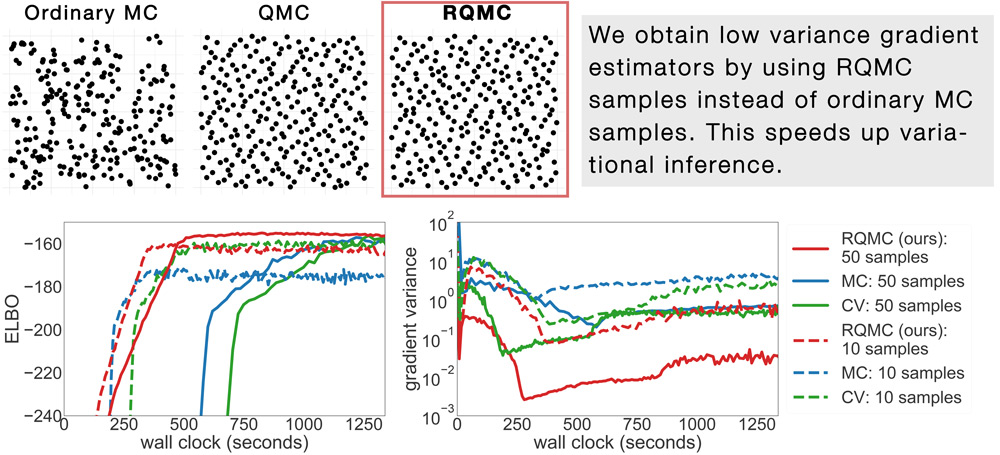# Quasi-Monte Carlo Variational Inference### Abstract

Many machine learning problems involve Monte Carlo gradient estimators. As a prominent example, we focus on Monte Carlo variational inference (MCVI) in this paper. The performance of MCVI crucially depends on the variance of its stochastic gradients. We propose variance reduc- tion by means of Quasi-Monte Carlo (QMC) sampling. QMC replaces N i.i.d. samples from a uniform probability distribution by a deterministic sequence of samples of length N. This sequence covers the underlying random variable space more evenly than i.i.d. draws, reducing the variance of the gradient estimator. With our novel approach, both the score function and the reparameterization gradient estimators lead to much faster convergence. We also propose a new algorithm for Monte Carlo objectives, where we operate with a constant learning rate and increase the number of QMC samples per iteration. We prove that this way, our algorithm can converge asymptotically at a faster rate than SGD. We furthermore provide theoretical guarantees on QMC for Monte Carlo objectives that go beyond MCVI, and support our findings by several experiments on large-scale data sets from various domains.

Type
Publication
International Conference on Machine Learning (ICML)
More
Oral Presentation
Date